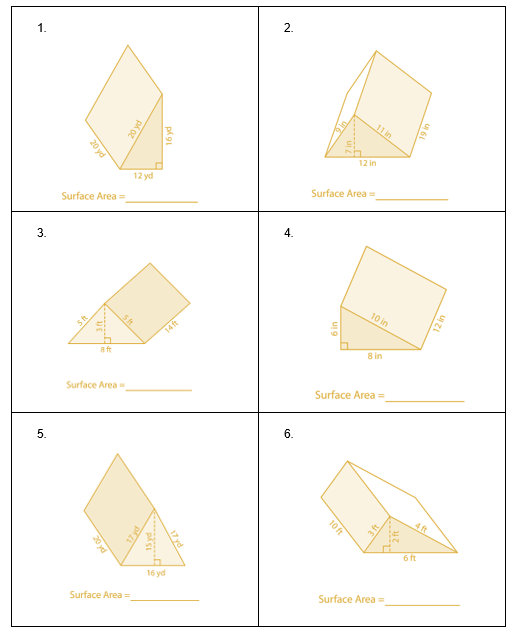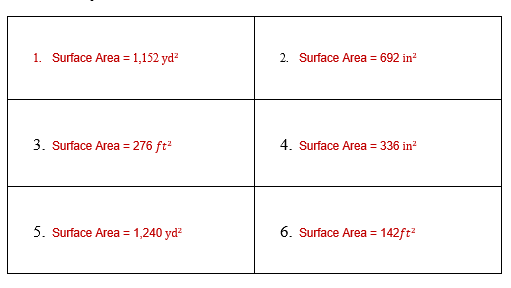# Surface Area of Triangular Prism Worksheet (with answer key + PDF)

A three-sided prism is called a triangular prism. It is a polyhedron with three faces joining corresponding sides, a triangular base, and a translated copy. A=ab+3bh, where a, b, and h are the prism’s side, base, and height, respectively, gives the surface area of a triangular prism.

## What is the “Surface Area of Triangular Prism Worksheet”?

This worksheet will explore some of the surface areas of the triangular prism.

## What is the Surface Area of a Triangular Prism?

The total of all the faces’ or surfaces’ areas is referred to as a triangular prism’s surface area. There are three rectangular and two triangular faces on a triangular prism. While the bases are referred to as the triangular faces, the lateral faces are described as being rectangular.

This worksheet will help the students to understand and find the surface area of the triangular prism. This worksheet helps students to visualize the net of a triangular prism made of three rectangles and two congruent triangles.

## Instructions on how to use the “Surface Area of Triangular Prism Worksheet.”

Worksheets on the Surface Area of Triangular Prisms explain the relationship between the Net and Surface Area of a Solid and provide students with concept-based practice questions. Study the concept and examples given and try to solve the given exercises below.

## Conclusion

The sum of the areas of all the triangle’s faces is the surface area. A triangular prism is a prism with three rectangular faces connecting its two congruent triangular faces. It consists of 5 faces, 6 edges, and 9 vertices.

If you have any inquiries or feedback, please let us know.

## Surface Area of Triangular Prism Worksheet (with answer key + PDF)

What is a Triangular Prism?

The pentahedron Triangular Prism has nine different nets. The bases’ vertices and edges are connected by means of their three rectangular sides. The rectangular sides of the triangular prism are joined to one another side by side. Every cross-section that is perpendicular to the base faces is a triangle. In contrast to the triangular prism, a triangular pyramid has four triangular bases that are joined together and all coincide with one another.

What is the Total Surface Area of a Triangular Prism?

A triangular prism’s total surface area is another name for its surface area. A triangular prism’s total surface area is equal to the sum of its faces’ surface areas. Three rectangular and two triangle faces make up a triangular prism. The bases are triangular faces, while the lateral faces are described as rectangular. When a triangular prism’s bases are arranged horizontally, the top and bottom (faces) of the prism are referred to as such. The surface area of a triangular prism is expressed in square units, like, m2, cm2, in2, ft2, etc.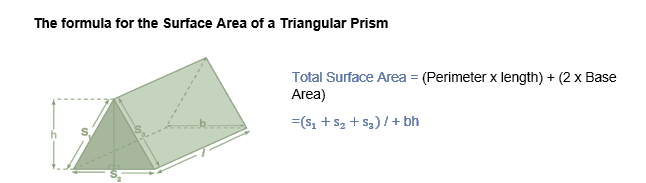### The formula for the surface area of a triangular prism is

Surface area = (Perimeter of the base × Length of the prism) + (2 × Base Area) = (S1 +S2 + S3) L + bh

where,

• b is the bottom edge of the base triangle,
• h is the height of the base triangle,
• L is the length of the prism and
• S1, S2, and S3 are the three edges (sides) of the base triangle
• (bh) is the combined area of the two triangular faces [2 × (1/2 × bh)] = bh

Lateral Surface Area of Triangular Prism

Any solid’s empty space between its bases is its lateral surface area. Thus, the base area is not taken into account when calculating the lateral surface area of a triangular prism. The lateral area is the region of the vertical faces when the bases of a triangular prism are oriented upward and downward.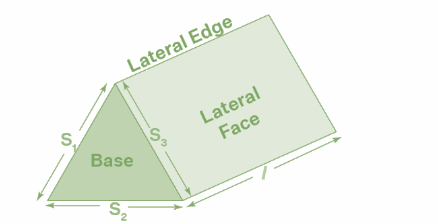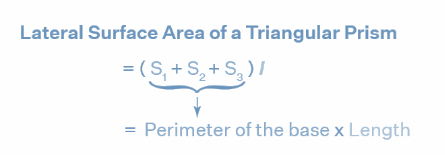Thus, the lateral surface area of a triangular prism is:

Lateral Surface Area = (S1 + S2 + S3) × l = (Perimeter × Length) or LSA = p × l

• l is the height (length) of a prism
• p is the perimeter of the base

How to Find the Surface Area of a Right Triangular Prism?

Example: Find the total surface area of a right triangular prism which has a base area of 60 square units, a base perimeter of 40 units, and a length of the prism is 7 units.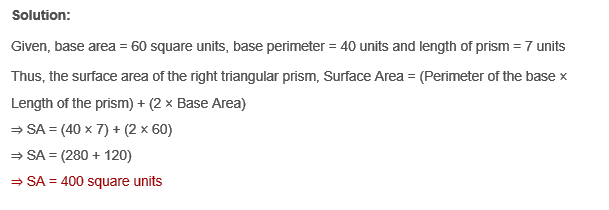## Worksheet

Find the surface area of each triangular prism.